GeeksforGeeks App
Open AppBrowser
Continue

## Related Articles

• RD Sharma Class 10 Solutions

# Class 10 RD Sharma Solutions – Chapter 16 Surface Areas and Volumes – Exercise 16.2 | Set 1

### Question 1. Consider a tent cylindrical in shape and surmounted by a conical top having height 16 m and radius as common for all the surfaces constituting the whole portion of the tent which is equal to 24 m. Height of the cylindrical portion of the tent is 11 m. Find the area of Canvas required for the tent.

Solution:

According to the question

Diameter of the cylinder = 24 m,

So the radius(r) = 24/2 = 12 m,

Height of the cylindrical part (H1) = 11m

and the total height of the shape = 16 m

So, the height of the cone (h)= 16 – 11 = 5m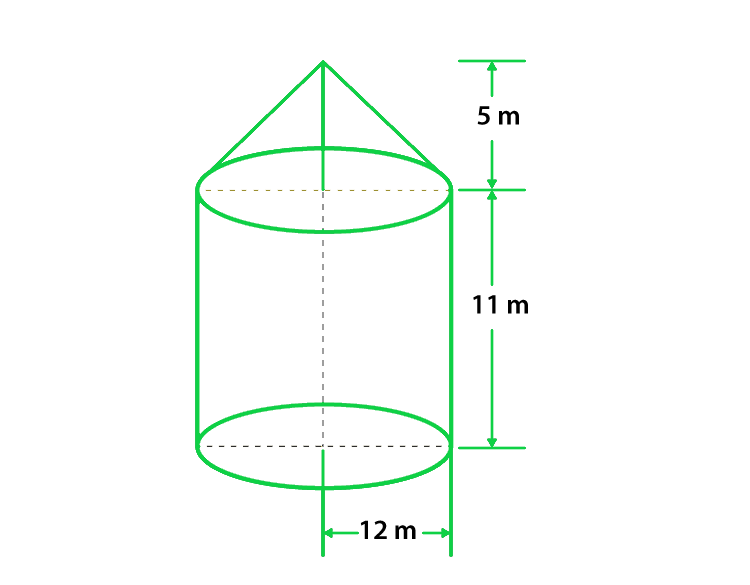Now, first we find the slant height of the cone

So, according to the formula of slant height

l = √r2 + h2

l = √122 + 52 = 13m

Now, we find the curved surface area of the cone

A1 = πrl

= 22/7 × 6 × 13  ……(1)

Now, we find the curved surface area of the cylinder

A2 = 2πrH1

= 2π(12)(11)  ……(2)

Now we find the total area of canvas required for tent,

So,

A = A1 + A2

= 22/7 × 12 × 13 + 2 × 22/7 × 12 × 11

= 490 + 829.38 = 1319.8 = 1320 m2

Hence, the total canvas required for tent is 1320 m2

### Question 2. Consider a Rocket. Suppose the rocket is in the form of a Circular Cylinder Closed at the lower end with a Cone of the same radius attached to its top. The Cylindrical portion of the rocket has radius say, 2.5 m and the height of that cylindrical portion of the rocket is 21m. The Conical portion of the rocket has a slant height of 8m, then calculate the total surface area of the rocket and also find the volume of the rocket.

Solution:

According to the question

Radius of the cylindrical part of the rocket(r) = 2.5 m,

Height of the cylindrical part of the rocket (h) = 21 m,

Slant Height of the conical surface of the rocket(l) = 8 m,

Now, we find the curved surface area of the cone

A1 = πrl

= π(2.5)(8)

= π x 20      ……(1)

Now, we find the curved surface area of the cone

A2 = 2πrh + πr2

= (2π × 2.5 × 21) + π (2.5)2

= (π × 10.5) + (π × 6.25)    ……(2)

Now we find the total curved surface area

A = A1 + A2

= (π x 20) + (π x 10.5) + (π x 6.25)

= 62.83 + 329.86 + 19.63 = 412.3 m2

Hence, the total curved surface area of the conical surface is 412.3 m2

Let us considered H be the height of the conical portion in the rocket,

So, l2 = r2 + H2

H2 = l2 – r2

h = √82 – 2.52 = 23.685 m

Now we find the volume of the conical surface of the rocket

V1 = 1/3πr2H

= 1/3 × 22/7 × (2.5)2 × 23.685     ……(3)

Now we find the volume of the cylindrical part

V2 = πr2h

V2 = 22/7 × 2.52 × 21

Therefore, the total volume of the rocket

V = V1 + V2

V = 461.84 m2

Hence, the total volume of the Rocket is 461.84 m2

### Question 3. Take a tent structure in vision being cylindrical in shape with height 77 dm and is being surmounted by a cone at the top having height 44 dm. The diameter of the cylinder is 36 m. Find the curved surface area of the tent.

Solution:

According to the question

Height of the tent = 77 dm,

Height of a surmounted cone = 44 dm,

Diameter of the cylinder = 36 m,

So, the radius of the cylinder(r) = 36/2 = 18 m

Therefore, the height of the cylindrical Portion(h) = 77 – 44 = 33 dm = 3.3 m

Let us considered l be the slant height of the cone,

So,l2 = r2 + h2

l2 = 182 + 3.32

l2 = 324 + 10.89 = 334.89 = 18.3 m

Now we find the curved surface area of the cylinder

A1 = 2πrh

= 2π184.4 m2      ……(1)

Now we find the curved surface area of the cone

A2 = πrh

= π × 18 × 18.3                ……(2)

Hence, the total curved surface of the tent

A = A1 + A2

Put all the values from eq(1) and (2)

A = (2π x 18 × 4.4) + (π x 18 × 18.3) = 1532. 46 m2

Hence, the total Curved Surface Area is 1532.46 m2

### Question 4. A toy is in the form of a cone surmounted on a hemisphere. The diameter of the base and the height of the cone are 6 cm and 4 cm, respectively. Determine the surface area of the toy.

Solution:

According to the question

The height of the cone (h) = 4 cm

Diameter of the cone (d) = 6 cm,

So, radius of the cone (r) = 3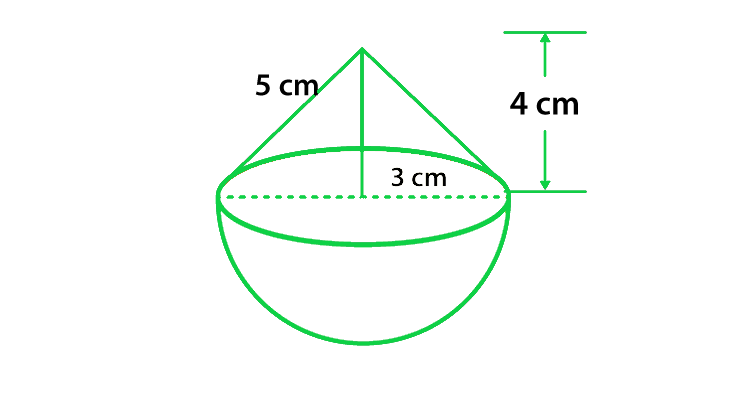Let us considered l be the slant height of the cone,

l = √r2 + h2

l = √32 + 42 = 5 cm

Now we find the curved surface area of the cone

A1 = πrl

= π(3)(5) = 47.1 cm2

Now we find the curved surface area of the hemisphere

A1 = 2πr2

= 2π(3)2 = 56.23 cm2

Hence, the total surface area of toy

A = A1 + A2

= 47.1 + 56.23 = 103.62 cm2

Hence, the curved surface area of the toy is 103.62 cm2

### Question 5. A solid is in the form of a right circular cylinder, with a hemisphere at one end and a cone at the other end. The radius of the common base is 3.5 cm and the height of the cylindrical and conical portions are 10 cm and 6 cm, respectively. Find the total surface area of the solid. (Use π = 22/7).

Solution:

According to the question

The radius of the common base (r) = 3.5 cm,

Height of the cylindrical part (h) = 10 cm,

Height of the conical part (h1) = 6 cm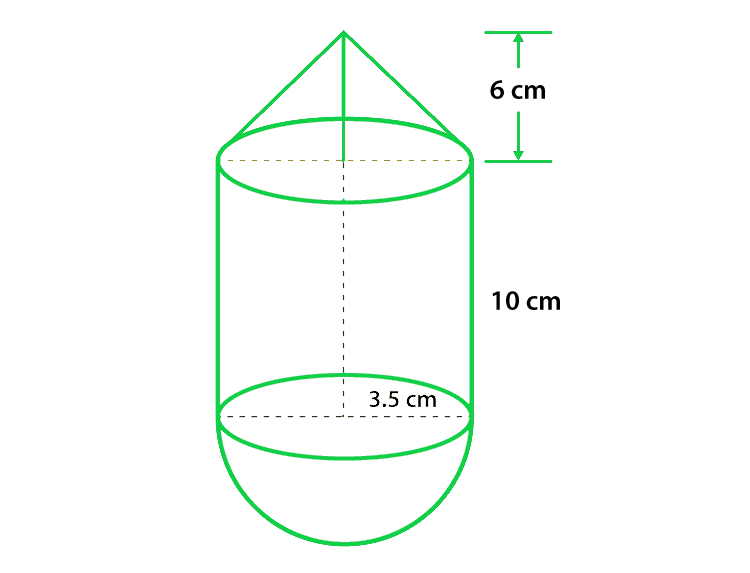Let us considered l be the slant height of the cone,

So,

l = √r2 + h2 = √3.52 + 62 = 48.25 cm

Now we find the curved surface area of the cone

A1 = πrl

= π(3.5)(48.25) = 76.408 cm2

Now we find the curved surface area of the hemisphere

A2 = 2πrh

= 2π(3.5) (10) = 220 cm2

Therefore, the total surface area of the solid

A = A1 + A2

= 76.408 + 220 = 373.408 cm2

Hence, total surface area of the solid is 373.408 cm2.

### Question 6. A toy is in the shape of a right circular cylinder with a hemisphere on one end and a cone on the other. The radius and height of the cylindrical parts are 5cm and 13 cm, respectively. The radii of the hemispherical and conical parts are the same as that of the cylindrical part. Find the surface area of the toy if the total height of the toy is 30 cm.

Solution:

According to the question

The height of the cylindrical part(h1) = 13 cm,

Radius of the cylindrical part(r) = 5 cm,

Height of the whole solid(H) = 30 cm,

The height of the conical part,

h2 = 30 – 13 – 5 = 12 cm

Now we find the slant height of the cone

l = √r2 + h22 = √52 + 122 = 13 cm

Now we find the curved surface area of the cylinder

A1 = 2πrh1

= 2π(5)(13) = 408.2 cm2

Now we find the curved surface area of the cone

A2 = πrl

= π(5)(13) cm2 = 204.1 cm2

Now we find the curved surface area of the hemisphere

A3 = 2πr2

= 2π(5)2 = 157 cm2

Hence, the total curved surface area of the toy

A = A1 + A2 + A3

= (408.2 + 204.1 + 157) = 769.3 cm2

Hence, the surface area of the toy is 769.3 cm2

### Question 7. Consider a cylindrical tub having radius as 5 cm and its length 9.8 cm. It is full of water. A solid in the form of a right circular cone mounted on a hemisphere is immersed in tub. If the radius of the hemisphere is 3.5 cm and the height of the cone outside the hemisphere is 5 cm. Find the volume of water left in the tub.

Solution:

According to the question

Radius of the Cylindrical tub(r) = 5 cm,

Height of the Cylindrical tub(h1) = 9.8 cm,

Height of the cone outside the hemisphere (h2) = 5 cm,

Radius of the hemisphere = 5 cm.

Now we find the volume of the cylindrical tub

V1 = πr2h1

= π(5)2 9.8 = 770 cm3

Now we find the volume of the Hemisphere

V2 = 2/3 × π × r3

= 2/3 × 22/7 × 3.53 = 89.79 cm3

Now we find the volume of the cone

V3= 1/3 × π × r2 × h2

= 1/3 × 22/7 × 3.52 × 5 = 64.14 cm3

Hence, The total volume

V = V2 + V3

V = 89.79 + 64.14 = 154 cm3

Hence, the total volume of the solid = 154 cm3

Hence, the volume of water left in the tube V = V1 – V2

V = 770 – 154 = 616 cm3

Hence, the volume of water left in the tube is 616 cm3.

### Question 8. A circus tent has a cylindrical shape surmounted by a conical roof. The radius of the cylindrical base is 20 cm. The height of the cylindrical and conical portions is 4.2 cm and 2.1 cm.  Find the volume of that circus tent.

Solution:

According to the question

Radius of the cylindrical part(R) = 20 m,

Height of the cylindrical part(h1) = 4.2 m,

Height of the conical part(h2) = 2.1 m,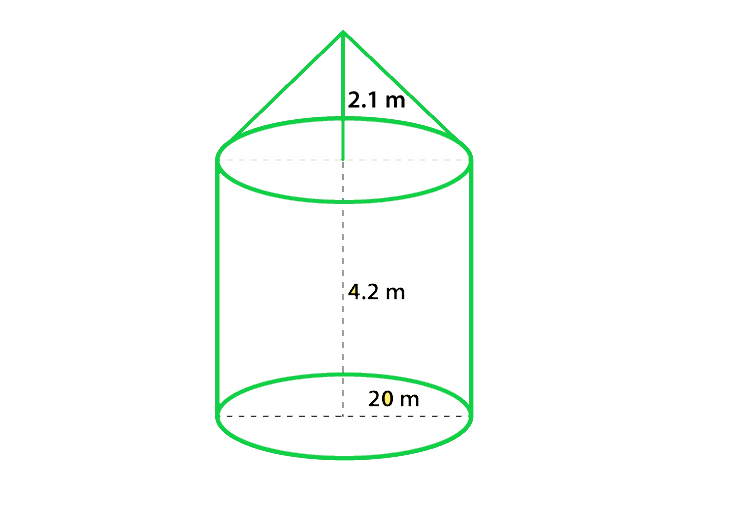Now we find the volume of the cylindrical part

V1 = πr2 h1

= π(20)2 4.2 = 5280 m3

Now we find the volume of the conical part

V2 = 1/3 × π × r2 × h2

= 1/3 × 22/7 × r2 × h2

= 13 × 22/7 × 202 × 2.1 = 880 m3

Hence, the total volume of the circus tent

V = V1 + V2 = 6160 m3

Hence, the volume of the circus tent is 6160 m3

### Question 9. A petrol tank is a cylinder of base diameter 21 cm and length 18 cm fitted with the conical ends, each of axis 9 cm. Determine the capacity of the tank.

Solution:

According to the question

Base diameter of the cylinder = 21 cm,

Radius (r) = diameter/2 = 25/2 = 11.5 cm,

Height of the cylindrical part of the tank (h1) = 18 cm,

Height of the conical part of the tank (h2) = 9 cm.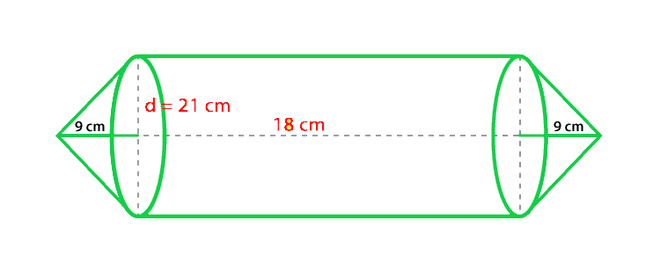Now we find the volume of the cylindrical portion

V1 = πr2 h1

= π(11.5)2 18 = 7474.77 cm3

Now we find the volume of the conical portion

V2 = 1/3 × 22/7 × r2 × h2

= 1/3 × 22/7 × 11.52 × 9 = 1245.795 cm3

Hence, the total the capacity of the tank

V = V1 + V2

= 7474.77 + 1245.795

= 8316 cm3

Hence, the capacity of the tank is 8316 cm3

### Question 10. A conical hole is drilled in a circular cylinder of height 12 cm and base radius 5 cm. The height and base radius of the cone are also the same. Find the whole surface and volume of the remaining Cylinder.

Solution:

According to the question

Height of the cone = Height of the cylinder = h = 12 cm,

Radius of the cone = Radius of the cylinder = r = 5 cm.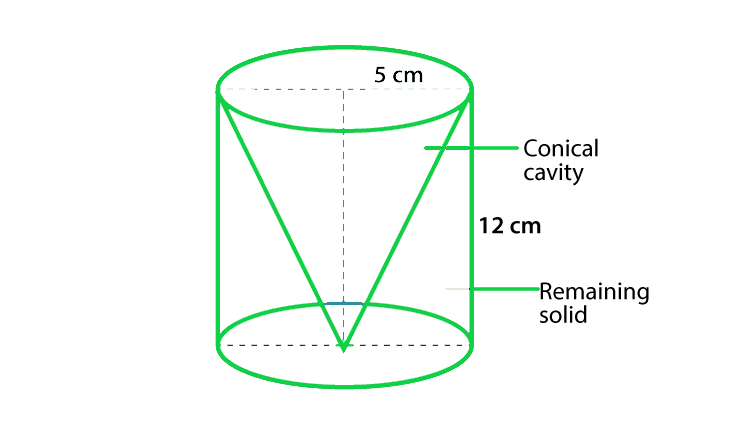Let us considered l be the slant height of the cone

So,

l = √r2 + h2 = √52 + 122 = 13 cm

Now we find the total surface area of the remaining part in the circular cylinder

A= πr2 + 2πrh + πrl

= π(5)2 + 2π(5)(12) + π(5)(13) = 210 π cm2

Now we find the volume of the remaining part of the circular cylinder

V = πr2h – 1/3 πr2h

= πr2h – 1/3 × 22/7 × r2 × h

= π(5)2(12) – 1/3 × 22/7 × 52 × 12 = 200 π cm2

Hence, the area of the remaining part is 210 π cm2 and the volume is 200 π cm2

### Question 11. A tent is in the form of a cylinder of diameter 20 m and height 2.5 m surmounted by a cone of equal base and height 7.5 m. Find the capacity of tent and the cost of canvas as well at a price of Rs.100 per square meter.

Solution:

According to the question

Diameter of the cylinder = 20 m,

So, the radius of the cylinder = 10 m,

Height of the cylinder (h1) = 2.5 m,

Radius of the cone = Radius of the cylinder = r = 15 m,

Height of the Cone (h2) = 7.5 m.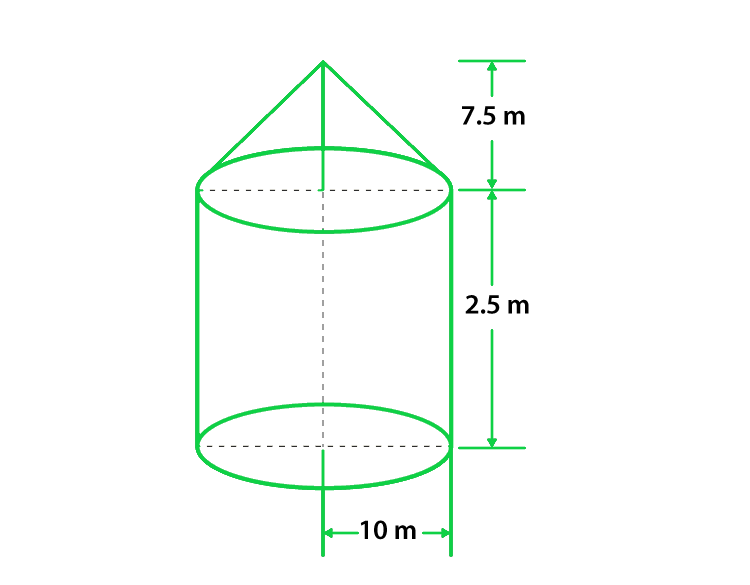Let us considered l be the slant height of the cone

So,

l = √r2 + h22 = √152 + 7.52 = 12.5 m

Now we find the volume of the cylinder

V1 = πr2h1

= π(10)2 2.5 = 250π m3

Now we find the volume of the Cone

V2 = 1/3πr2h2

= 1/3 × 22/7 × 102 × 7.5 = 250π m3

Hence, the total capacity of the tent

V = V1 + V2

= 250 π + 250 π = 500π m3

Hence, the total capacity of the tent = V = 4478.5714 m3

Now we find the total area of the canvas required for the tent is

S = 2πrh1 + πrl

= 2(π)(10)(2.5) + π(10)(12.5) = 550 m2

Hence, the total cost of the canvas is (100 x 550) = Rs. 55000

### Question 12. Consider a boiler which is in the form of a cylinder having length 2 m and there’s a hemispherical ends each of having a diameter of 2 m. Find the volume of the boiler.

Solution:

According to the question

Diameter of the hemisphere = 2 m,

So, the radius of the hemisphere (r) = 1 m,

Height of the cylinder (h) = 2 m,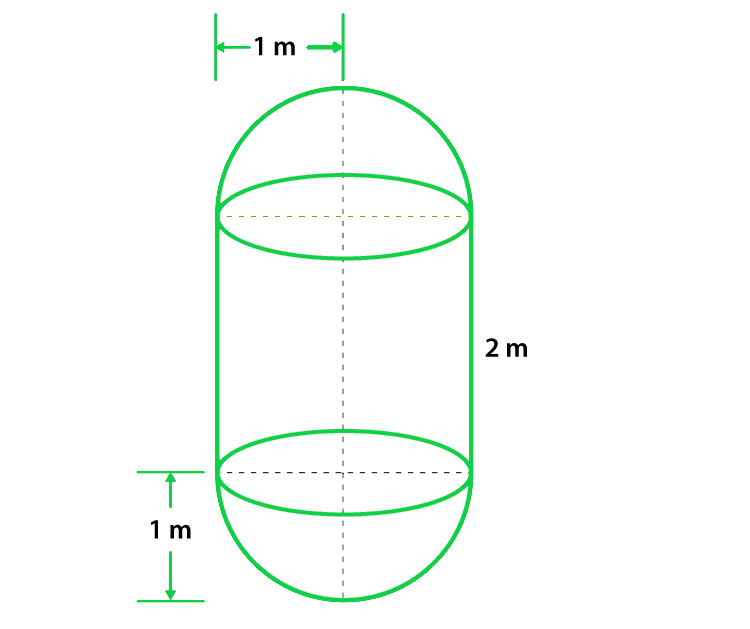Now we find the volume of the cylinder

V1 = πr2h

= π(1)2 x 2 = 22/7 × 2 = 44/7m3

Since, at each of the ends of the cylinder, two hemispheres are attached.

so, the volume of two hemispheres

V2 = 2 × 2/3πr3

= 2 × 2/3 × 22/7 × 13 = 22/7 × 4/3 = 88/21 m3

Hence, the volume of the boiler is

V = V1 + V2

V = 44/7 + 88/21 = 220/21 m3

Hence, the volume of the boiler is 220/21 m3

My Personal Notes arrow_drop_up
Related Tutorials